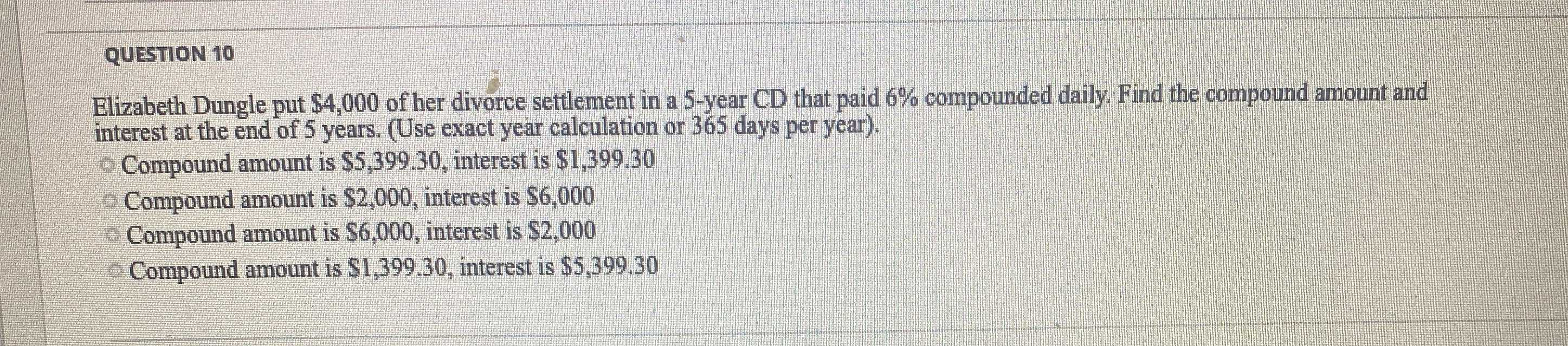### Still have math questions?

Algebra
QuestionElizabeth Dungle put $$\ 4,000$$ of her divorce settlement in a $$5$$ -year CD that paid $$6 \%$$ compounded daily. Find the compound amount and interest at the end of $$5$$ years. (Use exact year calculation or $$365$$ days per year).

Compound amount is $$\ 5,399,30 ,$$ interest is $$\ 1,399.30$$

Compound amount is $$\ 2,000$$ , interest is $$\ 6,000$$

Compound amount is $$\ 6,000 ,$$ interest is $$\ 2,000$$

Compound amount is $$\ 1,399.30$$ , interest is $$\ 5,399,30$$

A = $5399.30 interest rate$1399.30